﻿ Nuclide Spectra of Activities of Thorium, Uranium Series and Application in Gamma-spectrometry of Point Technogenic Samples

### Nuclide Spectra of Activities of Thorium, Uranium Series and Application in Gamma-spectrometry of Po...

Pop O.M., Simulik V.M., Stets M.V.

International Journal of Physics

## Nuclide Spectra of Activities of Thorium, Uranium Series and Application in Gamma-spectrometry of Point Technogenic Samples

Pop O.M.1, Simulik V.M.1,, Stets M.V.1

1Institute of Electron Physics of the National Academy of Sciences of Ukraine, Uzhgorod, Ukraine

### Abstract

The radioactive nuclei decay of the nuclides in the 232Th, 235U and 238U series is under consideration. The Bateman-Rubinson system of differential equations is applied. The activity of any n-th nuclide of the series has been calculated. The standard nuclide spectra are found and are compared with experimental nuclide spectra of activities. The results of the measurements are the time parameters, which determine the event date. The examples of the measurement of the experimental nuclide spectra in the nuclear gamma spectrometry of the point technogenic samples have been considered.

### Cite this article:

• Pop O.M., Simulik V.M., Stets M.V.. Nuclide Spectra of Activities of Thorium, Uranium Series and Application in Gamma-spectrometry of Point Technogenic Samples. International Journal of Physics. Vol. 4, No. 2, 2016, pp 37-42. http://pubs.sciepub.com/ijp/4/2/3
• O.M., Pop, Simulik V.M., and Stets M.V.. "Nuclide Spectra of Activities of Thorium, Uranium Series and Application in Gamma-spectrometry of Point Technogenic Samples." International Journal of Physics 4.2 (2016): 37-42.
• O.M., P. , V.M., S. , & M.V., S. (2016). Nuclide Spectra of Activities of Thorium, Uranium Series and Application in Gamma-spectrometry of Point Technogenic Samples. International Journal of Physics, 4(2), 37-42.
• O.M., Pop, Simulik V.M., and Stets M.V.. "Nuclide Spectra of Activities of Thorium, Uranium Series and Application in Gamma-spectrometry of Point Technogenic Samples." International Journal of Physics 4, no. 2 (2016): 37-42.

 Import into BibTeX Import into EndNote Import into RefMan Import into RefWorks

12
Prev Next

### 1. Introduction

Investigated samples can be considered as a chemical systems, i. e. as an ordered set of nuclides (chemical elements). Chemical systems are the generalization of the geochemical, biochemical and other nuclides systems in the samples. The sets of nuclides of the series 232Th, 235U and 238U belong to such systems as well. For such systems the methods of the nuclear gamma spectrometry of environment with semiconductor detectors are used.

The gamma spectrometry of the sample results in the table of activities A of the gamma-active nuclides. It can be presented graphically in the form of the experimental nuclide spectrum of the nuclide activity A . A comparative geochemical analysis on the basis of nuclides spectra, in which the nuclides 238U, 234U, 230Th, 226Ra (series 238U); 232Th, 228Ra, 228Ac (series 232Th) for different samples are presented, is enough informative. Nevertheless, the nuclide spectra were not used for the dating and other time parameters determining.

The methods of nuclear chronometers (the method of uranium series) for the dating and samples age determining are used. Such methods are based on the application of the equation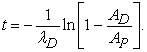(1)

Note that such methods are valid only for the closed chemical systems of nuclides.

These methods relate the activities of the parent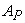and the daughter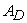nuclides;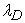is the decay constant. It is, e. g., a pair of long-lived nuclides AD 234U and AP 238U; AD 230Th and AP 234U; AD 231Pa and AP 235U. The equation (1) for the nuclide couple’s activities calculations is found after a number of assumptions on the basis of the Bateman equations [2, 3].

Note that a detail derivation of the equation (1) is presented in [4, 5]. Its analysis and mathematical backgrounds are beyond this brief article. The examples and problems of the nuclear chronometers methods application are considered in many investigations, see, e. g., [1, 4, 5, 6, 7, 8].

The evident increasing and complication of problems, e. g. the identification of the technogenic materials with the radioactive nuclides of the 232Th, 235U and 238U series, puts forward new demands to the existing methods.

Our investigation is the search for further development of the gamma spectrometric variant of the nuclear chronometers method. This study is based on the measurement of the experimental nuclide spectra of activities of any nuclides of the series 232Th, 235U and 238U. The results of its measurement, in particular, are the time parameters that determine the date of the event in the samples. The event here is the registered changes of the nuclide content of the 232Th, 235U and 238U series .

### 2. The Solutions of the Bateman-Rubinson Equations. The Standard Set of Nuclides

Consider briefly the algorithm of the standard set method. The activity Аn(Тe) of any n-th nuclide of the series can be presented by the generalized for the case of branched chains expression, which is the solution of the Bateman-Rubinson system of differential equations [9, 10, 11, 12, 13], and has the form: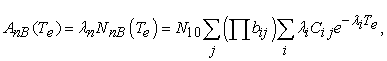(2)

where А10 are the initial values of the parent nuclei activity; bij are the branching coefficients («internal» branching ratios, which values are the nuclear constants);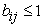; Сіj are the coefficients, which depends on decay constants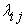, Те is the proper time, which is present in the activities calculations.

The calculations are performed for:

- one mole of a substance: N10 = NА = 6,022.1023 nuclei (Avogadro's number);

- the proper time Те 232Th (from 0 to 3,17.1012 years); 235U (from 0 to 3,17.1012 years); 238U (from 0 to 3,17.1012 years).

The nuclear data are taken from . Branching ratios bi with the formation of clusters are not considered.

The following conditions are chosen for solving of the Bateman-Rubinson system of differential equations:

- the activities of all nuclides except parent (activity А10) in the proper time moment Те=0 are taken equal to zero;

- for an arbitrary time moment Те any other possibilities of nuclei entrance and losses except the processes of decay and formation are absent.

A set of all these conditions, of the analytical expressions (2) and theirs solutions are called the standard conditions.

An ordered set of all radionuclides of one series, which activities А(Tе) are determined by the expression (2), we call the standard set of this series. The standard nuclide set (SNS) is the ordered list of one series nuclides that are linked genetically by the mutual decay and formation . The standard nuclide spectra of activities are the quantitative characteristic of the standard sets.

The result of event, as was indicated, is the registrated change of the nuclide content in the sample. It leads to the standard nuclide sets formation of the new daughter series . We called these sets the standard daughter nuclide sets. The result of their formation will be the non-standard sets appearance. The non-standard set is the composition (sum) of two (and more) standard sets, which formation is caused by the event.

The experimental nuclide sets it is the nuclear gamma spectrometry registrated manifold of the parent and daughter 232Th, 235U and 238U nuclide series in the samples. The standard and non-standard sets can be their models.

The experimental nuclide spectra of activities are the quantitative characteristics of the experimental nuclide sets. Indeed, the experimental nuclide spectra it is the ordered sequence of the experimental values of the gamma activities of the А nuclides of the 232Th, 235U and 238U sets.

2.1. Tables of Standards

We have found the time dependence of the activities A=f(Te) for all nuclides of the 232Th, 235U and 238U series. For these purposes the calculations of the values of the activities in (2) for the enough big number of points of the proper time Те is applied. The table found is recalculated to the experimental values of the activities А. The table of standards is obtained as a result. Any row of this table is the standard nuclide spectrum of activities that is the standard for the concrete value of the proper time Те. The values of activities for the corresponding nuclide series are given in the columns of the table.

2.2. The Measurement of the Experimental Nuclide Spectrum of Activities in the Table of Standards

The measurement is in comparison of the experimental nuclide spectrum ENS (Tm) with the corresponding standard that is the standard nuclide spectrum SNS (Te):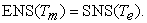(3)

The experimental nuclide spectrum corresponds to the standard conditions if the equality (3) is valid.

Measuring the gamma activities of scales we are working in the time scale Tm. It is the date (an ordinary calendar time). The calculations of the nuclide gamma activities are fulfilled in the scale of proper time Te. The comparison (3) put into correspondence the proper time Te to the date time Tm. The value of this proper time Те is interpreted as the age (the duration of the existence) of the standard nuclide set, which is formed as a consequence of the event.

However, the nuclide activities, which form an experimental nuclide spectra, are the experimental values. These values are not determined in some sense. Moreover, the experimental set can be a nonstandard set. Therefore, the standard nuclide spectrum, which is equal exactly to the experimental nuclide spectrum, cannot be found. Thus, for the activity Аnm) of n–th nuclide the necessity of taking into account the uncertainties results in the inequality: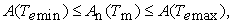(4)

where А(Теmin) belongs to the standard SNS А(Теmin); А(Теmax) belongs to the standard SNS А(Теmax); Аnm) belongs to the experimental nuclide spectrum ЕNS (Tm).

Since SNS А(Теmin) and SNS А(Теmax) are the standard spectra then the experimental nuclide spectrum ENS (Tm) satisfies the standard conditions as well.

By choosing the SNS A(Теmin) and the SNS A(Теmax) from the table of standards, we choose the meaning of the times Теmin and Теmax. Thereat, the time interval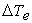and its mean value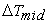.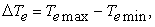(5)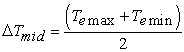(6)

are determined. The value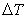can be considered not only as an uncertainty of time Те, but as some “interval of standardization” of the experimental nuclide spectrum ENS (Tm) as well. Inside this intervalthe experimental set is considered as the standard set.

2.3. Decomposition of the Experimental Nuclide Spectra

In order to determine the parent nuclide spectrum PNS or daughter nuclide spectrum DNS it is necessary to fulfill the decomposition of the experimental nuclide spectrum ENS. The nuclide spectra peculiarity is as follows. It is the result of term by term subtraction of the corresponding eponymous activities of the experimental and standard spectra.

In this case one can write: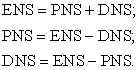Procedure of decomposition allows to find the standard sets on the basis of the experimental spectrum. Moreover, it allows to determine their standard nuclide spectra and theirs time coordinates Tе in the tables of standards. After the decomposition one of the known method of nuclear chronometers can be used for the determination of the proper time Tе.

Therefore, the pairs of nuclides, which are used in the methods of nuclear chronometers (1), contains in the standard set of all nuclides of the series. Thus, the standard nuclide set as a whole can be considered independently in the role of nuclear chronometer.

### 3. The Experimental Part

In this article the data for a few point technogenic samples are presented. These samples have a low but enhanced (in comparison with natural) activity. (The samples with higher, in comparison with natural, activity.) The radioactive source is called point if its size parameters (e. g. height h and radius r) are essentially lower (in 5-10 times) from the corresponding parameters of the detector (h = 60 mm; r = 50 mm). Such point-like conditions were used in the selection of the samples. Choosing the point samples we eliminated the problems of self-absorption, standard selection etc., during the samples gamma-spectroscopy.Download asVeiw figureFigures index
Figure 1. The characteristic apparatus gamma-spectrum of the technogenic sample

Apparatus gamma-spectra of technogenic samples found at HPGe-detector are 150 sm3 (energy resolution – 2 KеV for the line 1332 KеV 60Co), and at Ge(Li)-detector are 100 sm 3 (energy resolution – 3,9 KеV for the line 1332 KеV 60Co). The passive multilayered low background detector defense is used. The samples are measured without any special preparation. The samples impermeability was guaranteed. The length of the measurement is 2–4 hours. The characteristic apparatus gamma-spectrum of the technogenic sample is given on the Figure 1.

### 4. Measurement Errors

Program package of SEG-40-Ge complex determines the absolute (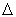А(Те)) and the relative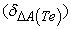measurement errors of the activities А(Те). The values of these errors are influenced at the determination of such important quantities as the time intervalvalues errors and errors due to decomposition.

The appearance of the time intervaland its value are the consequences of the different types measurement errors and uncertainties. The values Теmin, Теmax from the formula (2) cannot be determine analytically. Therefore, the assertion is accepted. For the monotonically increasing (decreasing) time dependences А(Те) the uncertainties of the times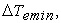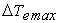are linearly proportional for the uncertainties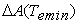,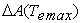. Further, it is accepted that relative errors of the activities А(Теmin) and times Те determination are equal, i. e.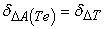. Therefore, if the relative errors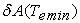and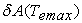are known, then the absolute errors can be determined in terms of these relative errors: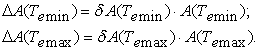The relative error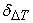of the time intervalvalue is given by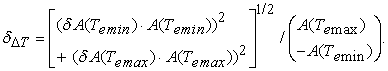The decomposition of spectra is the term by term subtraction of the experimental nuclide spectrum activities Аn(Тm) from the eponymous activity of some standard Аnе(Тm):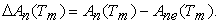The value Аn(Тm) is the experimental quantity. The value А(Тm) is the calculating quantity, which is determined on the basis of its parent nuclide activity. The relative error of the value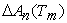determination is given by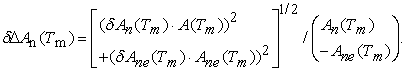For the problems, where the decomposition is necessary, the found value of the relative error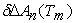will be taken into account in the formulae for the errorof time intervaldetermination.

In the method of the nuclear chronometers the formula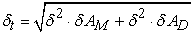is used for the relative time error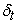evaluation. Here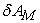,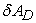are the relative errors of the corresponding activities determination.

### 5. The Results of the Investigation

The standard sets determination includes the determination of the experimental nuclide spectra of activities ENS А(Тm) in the table of standards. It finds a graphic mapping, respectively.

Figure 1 gives experimental nuclide spectrum ENS of А sample 1th and standards of comparison SNS А(Теmin) and SNS А(Теmax), which satisfy the condition (4). Experimental nuclide spectrum of the sample represents the standard nuclide set of the series 232Th. Fig. 3 shows the positions of the experimental nuclide spectrum ENS А and the standards of comparison SNS А(Теmin) and SNS А(Теmax) at the nuclide activities time dependence of the series 232Th. Imaginary they look like the points of intersection of the vertical line with these dependences and are presented as the rectangle. The width of «the rectangle» is given by the value of the time interval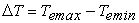. This interval represents the uncertainty of the position of the experimental nuclide spectrum ENS А given by the formula (4). The given measurement errorsare found in condition that the activity determination error is equal to 5%.Download asVeiw figureFigures index
Figure 2. The experimental nuclide spectrum of activities of the sample 1th (circles – dashed line), and the standard spectra SNS A(Теmin) (four squares) and SNS A(Теmax) 232ТhDownload asVeiw figureFigures index
Figure 3. Time dependence of the activities of the standard nuclide set of the series 232Тh (1 – 232Th, 2 – 228Ra, 228Ac, 3 – 228Th, 224Ra, 4 – 220Rn, 216Po, 212Pb, 212Bi, 5 – 212Po, 6 – 208Tl) of the sample 1th, time interval(in the form of the rectangle) and Тmid (dashed line in the rectangle)

Figure 4 shows experimental nuclide spectrum ENS А of the sample 10s and the comparison standards SNS А(Теmin) and SNS А(Теmax). Given sample contains the material, which contains the noticeable activities of the daughter nuclides 238U – 230Th, 226Ra, 214Pb, 214Bi. Activities of the parent 238U and daughter 234Th are relatively smaller. Therefore, the sample contains a nonstandard set. It is a composition of two standard sets: the standard set 238U and the standard set 230Th. We fulfill the decomposition, by selecting at first the standard set 238U, and after that the standard set 230Th: DNS 230Th = ENS A(Те) – PNS 238U. The result after decomposition is given by the Figure 5.Download asVeiw figureFigures index
Figure 4. The experimental nuclide spectrum of activities of the sample 10s (circles – dashed line) and the standard spectrum SNS (four squares) 238U before the decompositionDownload asVeiw figureFigures index
Figure 5. The experimental nuclide spectrum of activities of the sample 10s (circles – dashed line), and the standard spectrum SNS A(Теmin) (four squares) and SNS A(Теmax) (rectangles) 230Th after the decomposition

The results of the measurement are given in the table. Moreover, the data for the nuclear chronometers method are given in the table as well. We determine, as it was already considered above, the time interval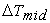(the formula (6)) of experimental set of the nuclides of the series. The time t (formula (1)) is determined in the method of nuclear chronometers. The conclusion about the satisfactory comparability between the values found with the help of two data methods can be suggested by the comparison of the valuesand t.

The experimental nuclide spectra of the samples 1th, 2th satisfy the condition (4). It means that the main component of their experimental sets are the standard sets 232Th.

The experimental nuclide spectra of the samples 3u – 10u satisfy the condition (4). The standard sets method data and the nuclear chronometers data testify about the satisfactory comparability between each other. The standard sets of 238U are the main parts of their experimental sets. Existence duration large values of the determined standard set of the 238U (107 – 108 years) allowed to suggest that they were formed enough long ago (maybe in the epoch of the intensive geologic processes) and were not changed from these times.

The experimental nuclide spectra of the samples 10s – 13s do not satisfy the condition (4). After the decomposition of the experimental sets the standard sets and nuclear chronometers methods data are comparable within the errors. Therefore, the result of the decomposition demonstrates that the experimental nuclide set of the samples 10s – 13s can be represented as the composition of the standard set of 238U and the standard nuclide set of 230Th.

Relatively large values of the measurement errors can be localized by the decreasing of the measurement duration.

#### Table 1. Comparison of the Standard Sets and the Nuclear Chronometers MethodsDownload asPowerPoint Slide

Veiw figure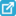View current table in a new window

### 6. Conclusions

The standard nuclide spectra of the nuclide activities А of series 232Th, 235U, 238U have been calculated. The table of standards by the nuclide activities А time dependence is created. Each spectrum from the table of standards is shown to be the standard itself. The experimental nuclide spectrum of activities А, by the way of its comparison with the standards of the standard nuclide spectrum А, has been measured. The measurement result is the time coordinate Те, which is the position from the experimental nuclide spectrum of activities А in the table of standards. The found value can be interpreted as the duration of the standard set existence. Here the duration of the existence is considered as the time interval started from the moment of the event, in which this event appeared, and finished in the moment of the measurement.

### Acknowledgement

The authors are much grateful to the director of the Institute of Electron Physics, full member of the Academy of Sciences of Ukraine O.B. Shpenik for the support of these investigations.

### References

  Titaeva, N.A., Nuclear geochemistry, Taylor Francis Inc, United States, 1994, 304.In article  Bateman, Η., “Solution of a system of differential equations occurring in the theory of radioactive transformations,” Proc. Cambridge Phil. Soc., 15. 423-427. 1910.In article  Rubinson, W., “The Equations of Radioactive Transformation in a Neutron,” Flux. J. Chem. Phys., 17 (6). 542-547. 1949.In article  Faure, G., Principles of isotope geology, 2-nd ed, John Wiley & Sons, 1986, 589.In article  Wagner, G. A., Age Determination of Young Rocks and Artifacts: Physical and Chemical Clocks in Quaternary Geology and Archeology, Springer Science & Business Media, 1998, 466.In article  Choppin, G. R., Liljenzin, J. O., Rydberg, J., Radiochemistry and Nuclear Chemistry, Butterworth-Heinemann, Woburn, 2001, 858.In article  Bourdon, B., Henderson, G. M., Lundstrom, C. C., and Turner, S. P., Uranium-series geochemistry, (Reviews in Mineralogy & Geochemistry, Vol. 52), Mineralogical Society of America, Washington, DC, 2003, 656.In article  Vértes, A., Nagy, S., Klencsár, Z., Lovas, R. G., and Rösch, F., Handbook of Nuclear Chemistry, Springer Science & Business Media, 2010, 2444.In article  Pop, O.M., Stets, M.V., “Models of evolution of the sets of radioactive 90Th232, 92U235, 92U238 nuclide series and their applications,” Reports of the National Academy of Sciences of Ukraine, 4. 65-71. 2013. (in Ukrainian).In article  Firestone, R.B., Shirley, V.S., Baglin, C.M., Table of isotopes CD-ROM. Eight Edition Version, 1, John Wiley & Sons, Inc., New York, 1996.In article  Pressyanov, D. S., “Short solution of the radioactive decay chain equations,” Am. J. Phys., 70 (4). 444-445. 2002.In article  Cetnar, J., “General solution of Bateman equations for nuclear transmutations,” Ann. Nucl. Energy, 33. 640-645. 2006.In article  Sun, Y., Buscheck, T. A., and Hao, Y., “An analytical method for modeling first-order decay networks,” Computers & Geosciences, 39. 86-97. 2012.In article  Thibes, R., and de Oliveira, S. L., “General solution to Bateman’s differential equations with direct index notation,” International Journal of Pure and Applied Mathematics, 93 (6). 879-883. 2014.In article